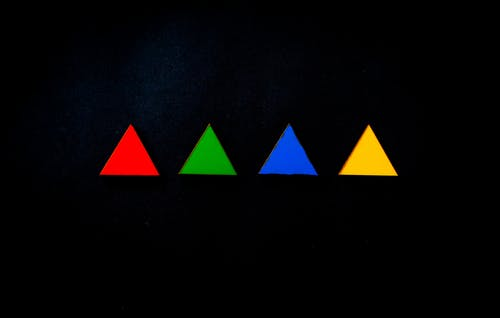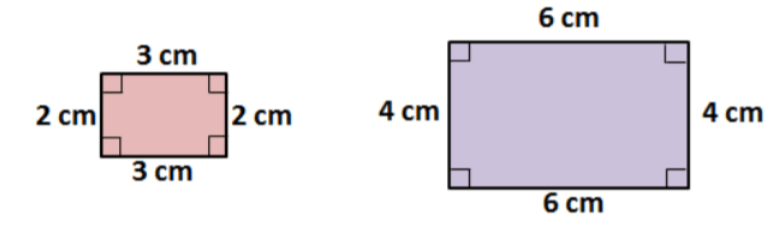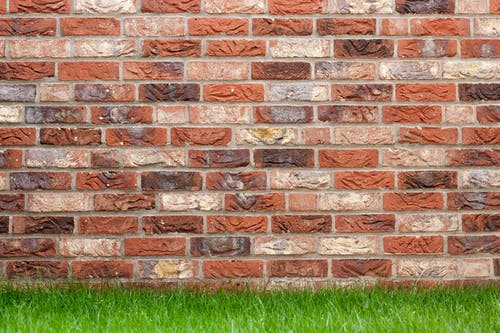# Teaching Congruent Figures

When learning about congruent figures in geometry, students learn how to label shapes with tick marks to show side and angle congruence. Though these beginning lessons on congruent figures may seem challenging for some students, teachers can help out in a variety of ways!

So if you’re teaching congruent figures, we bring you a few teaching tips that are guaranteed to keep your students engaged throughout the lesson! Read on and learn more.## Tips on Teaching Congruent Figures

### What Is a Figure?

Start your lesson by explaining what we mean by “figure” in geometry. Define a figure as a set of points in a closed plane or space. A figure can be 2-dimensional if it is in a plane and 3-dimensional if it is in space.

Add that two figures are said to be similar if they have the same shape but not the same size.

Explain that the corresponding angles of the two similar figures are equal, and the corresponding sides have the same ratio.

Present an example on the whiteboard of two figures that are similar, such as the figures below:Ask students to reflect on why these two figures are similar. Do they have the same size? No. The first figure is smaller than the second one. Do they have the same shape? Yes. Both of them are rectangles, even though the size differs.

### What Are Congruent Figures?

Now that you’ve defined figures and similar figures, you can move on to explaining what we mean by “congruent figures”. Simply put, congruent figures are figures that have the same shape and the same size.

The corresponding angles of two congruent figures are equal. The corresponding sides are equal. Present an example on the whiteboard of two figures that are congruent, such as the figures below:Ask students to think about why these two figures are congruent. Do they have the same shape? Yes, both of them are pentagons. Do they have the same size? Yes, their size is identical.

### Launch a Video Lesson!

If you can, include a few videos in your lesson on congruent figures to make it more visually appealing. For instance, you can play this video that explains what are congruent figures, when two figures are said to be congruent, and includes examples of congruent figures (polygons, segments, etc.)

Then, proceed by showing this video that features a brief explanation of congruent and noncongruent figures, followed by a brief cartoon where Congruent Island is attacked by the Dilation Pirates, who threaten to make all the inhabitants a different size and shape.

Then, play this video that presents the difference between similar and congruent figures. The video contains a plethora of everyday figures as examples, such as cars, shoes, fish, etc., that children will find easy to relate to.

Finally, end your lesson with this fun song on congruent shapes that children are guaranteed to enjoy. With its relatable examples as brick walls, floor tiles, and car tires, and its cute rhymes, students will learn facts about congruence that stick!

## Activities to Practice Congruent Figures### Congruent and Similar Figures Game

This online game will help students improve their skills of quickly identifying whether two or more figures are congruent or similar. To implement this activity in your classroom, make sure there’s a suitable device for each child.

Pair students up and provide instructions. Explain that they will play a game where they will be presented with one figure and asked to identify which other figures are either congruent or similar to the given figure.

For each correctly identified similar or congruent figure, players earn points. In the end, students compare their scores with the other person in their pair. The student with the highest score wins the game. Homeschooling parents can use the game as an individual activity.

### Congruent Tug of War

This game will help students sharpen their skills at recognizing which figures are congruent and which aren’t. To play this game in your classroom, make sure that there are a sufficient number of devices (in this game, you’ll need one computer per two students).

Pair students up and provide instructions. Use the row of number keys above the letters on the keyboard. One student is the red team and uses the keys 1 to 5, whereas the other one is the blue team and uses the keys 6 to 0.

Explain to students that the aim is to pull the yellow marker on the tug of war rope toward them. Every time a player answers a question correctly, the marker will move toward them. If they answer incorrectly, the marker moves toward the other player.

The winner is the player that will manage to have the marker closest to them when the game ends. Even though this is a group activity, homeschooling parents can also use the game with their child, for instance by asking the older ones to join in.

### Congruent Figures Matching Game

This is a fun, matching game that will help students reinforce their knowledge of congruent figures. To implement this game in your classroom, you’ll need construction paper, scissors, a pencil, as well as a timer.

Draw a variety of figurines on the construction paper, such as polygons, triangles, circles, etc., and cut them. Create several piles of these. Make sure you have plenty of same-size and same-shape figures in each pile, as well as figures that are the same shape, but a different size.

Divide students into groups of 3 or 4 and provide a pile to each group. The piles given to each group should be identical. Explain to students that they should work with the other members of their group in order to find and match the congruent figures as fast as they can.

This game is all about speed. Place a timer in the middle of the classroom and provide a few minutes for this activity. When the time is up, go around each group and count the number of matched congruent figures. The group with the highest number of matches wins the game.

## Before You Leave…

If you liked our tips on teaching congruent figures, and you’re looking for more math resources for kids of all ages, sign up for our emails to receive loads of free resources, including worksheets, guided lesson plans and notes, activities, and much more!

Feel free to also head over to our blog, where you’ll find plenty of free and awesome resources! And if you’d like to become a member, join us in the Math Teacher Coach community!